Intuitionistic Logic Explorer < Previous   Next > Nearby theorems Mirrors  >  Home  >  ILE Home  >  Th. List  >  ax11o Unicode version

Theorem ax11o 1744
 Description: Derivation of set.mm's original ax-11o 1745 from the shorter ax-11 1438 that has replaced it. An open problem is whether this theorem can be proved without relying on ax-16 1736 or ax-17 1460. Normally, ax11o 1744 should be used rather than ax-11o 1745, except by theorems specifically studying the latter's properties. (Contributed by NM, 3-Feb-2007.)
Assertion
Ref Expression
ax11o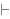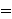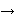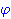Proof of Theorem ax11o
Dummy variable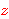is distinct from all other variables.
StepHypRef Expression
1 ax-11 1438 . 221ax11a2 1743 1Colors of variables: wff set class Syntax hints:wn 3wi 4wal 1283 This theorem was proved from axioms:  ax-1 5  ax-2 6  ax-mp 7  ax-ia1 104  ax-ia2 105  ax-ia3 106  ax-in2 578  ax-io 663  ax-5 1377  ax-7 1378  ax-gen 1379  ax-ie1 1423  ax-ie2 1424  ax-8 1436  ax-10 1437  ax-11 1438  ax-i12 1439  ax-4 1441  ax-17 1460  ax-i9 1464  ax-ial 1468 This theorem depends on definitions:  df-bi 115  df-nf 1391  df-sb 1687 This theorem is referenced by:  ax11b  1748  equs5  1751
 Copyright terms: Public domain W3C validator